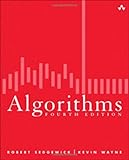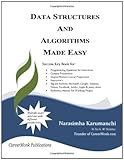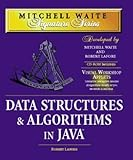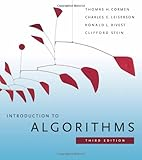# 5 Best Algorithms Books For Software DevelopersThe field of computer science has evolved into the most adaptive industry of the modern world. Every industry relies on computers for data processing, operation handling and data mining activities. Different operations are performed with the help of complex programs, which are based on algorithms. An algorithm can be defined as a step-by-step procedure used for solving a particular problem. These are the key elements in data processing applications, calculation and automated reasoning applications. If you are planning to be an efficient programmer or web application architect, you need to understand algorithms in an effective manner.
An understanding of algorithms demands a high-level understanding of mathematics. You have to be well-versed with numerical analysis for writing new algorithms and understand existing ones. There are several methods to solve a numerical problem and same thing holds true for algorithms. It is important to choose the most efficient algorithm for any particular problem. Most of the leading software and IT companies lay special attention on understanding of algorithms.
If you are new to algorithms, here are some excellent books to help you through the process.

## Algorithms(By: Robert Sedgewick and Kevin Wayne )

This book is designed for every programmer and covers the most important algorithms that every programmer should know. The differentiating feature of this book is the source code that can be used for real-time problem solving. All the implementations in this book are simple to understand and motivate readers towards practical implementation of algorithms. Readers are offered every single detail and learn from the practical problems given in the book. Every chapter contains exercises and experiments to help you sharpen your skills.

## Data Structures and Algorithms Made Easy: Data Structure and Algorithmic Puzzles(By: Narasimha Karumanchi )

If you have some basic knowledge of algorithms, this book is perfect for you. This book consists of all the important algorithms that one might learn through any university course. The book is written in an easy to understand manner and offers detailed concepts. You can learn the concept of data structures and algorithms from scratch. If you are preparing for an interview, this book is the best resource available over the internet. It will help you revise all the concepts of data structures and algorithms with proper examples.

## Data Structures and Algorithms in Java(By: Robert Lafore )

Data structures and algorithms are among the most difficult aspects of computer science, but this book makes it fun and easy to learn process. It starts with basic data structures including array, stacks, trees, queues, hash tables, sorting, searching, and recursion. This book suits the requirement of every programmer from beginning to the advanced stage. Every concept is explained in detail with examples and its real-world implementation. The core idea of the book is to help you understand data structures, algorithms and improve the performance of an algorithm.

## Introduction to Algorithms(By: Thomas H. Cormen , Charles E. Leiserson , Ronald L. Rivest, Clifford Stein )

Algorithms are much more than computation steps that help in finding solution to a given problem. You need a deep understanding of algorithms for efficient programming and that is what this book helps you to learn. This book is designed for programmers with an experience in algorithm handling and good knowledge of mathematics. You will understand concepts like the factors that define efficiency of an algorithm, reason behind the efficiency, selection of data structures for particular problems and best tips to handle those data structures. You would require some experience to get most out of this book.

## The Algorithm Design Manual(By: Steven S Skiena )

The key difference between this book from other algorithm materials is the emphasis of real-world implementation of algorithms. Unlike other books with a mathematical approach, this book helps in understanding the concept in an easy language. Every algorithm is explained with the help of real life problems and the second half of the book focuses on problems itself. If you are a programmer, you can find every possible problem that you would every encounter, within this book.

If you are a programmer, algorithms are an important part to learn. The key is to understand the real-world implementation of algorithms instead of their mathematical proof. Algorithms are an important part of every job interview and a better understanding will help you crack your interviews. Make sure to prepare multiple examples of these algorithms for your interview. You need to work on mathematical concepts for learning and devising efficient algorithms.

•••